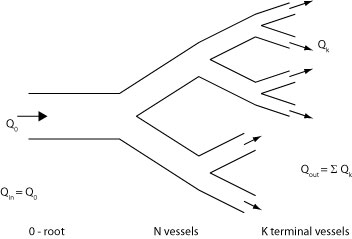# the new definition of reservoir pressure

 The new definition of the reservoir pressure is not based directly on the detailed solution provided by the method of characteristic solution discussed in the wave intensity pages. To provide a more tractable theory, we consider the arterial system to be made up of a network of N vessels. We assume that one of the vessels 0 is connected to the ventricle and that there are K terminal vessels connected to the microcirculation. We then define a time-varying average pressure in each vessel Pn(t) as the spatial integral over the length of the artery. This is shown in the sketch to the right where N=11, K=6 with the termini indicated by arrows going out of the system and the inlet to the network 0 is indicated by the arrow directed into the system. The conservation of mass for the arterial network is simply that the rate of change of the net volume V of the system is equal to the difference between volume flow rate into the the root Q0 and the net flow out of the terminal vessels Qout.dV/dt = Q0 - Qout

where the sum is taken over all of the vessels. If we also assume that the resistance at the end of each terminal vessel is resistive with resistance Rk, then the flow out of the kth terminal vessel can be written

Qk = (P - P)/Rk

where P is the pressure at which flow through the microcirculation ceases. It can be greater than the venous pressure because of waterfall effects and we assume that it is the same for all termini. We also assume that the compliance of the nth vessel is Cn. The mass conservation equation can now be written in terms of the properties of the individual vessels

ΣN Cn dPn/dt = Q0 - ΣK (Pk - P)/Rk

where ΣN is the sum over all of the vessels and ΣK is the sum over the terminal vessels.

### The Windkessel pressure

To illustrate the similarities (and the differences) between the classical Windkessel pressure and the reservoir pressure, we will show how the Windkessel pressure can be obtained in this formulation.

The essential assumption about the Windkessel pressure PWk is that it is uniform throughout the arterial system. With this assumption, the pressure can be taken outside of the summations and the mass conservation equation reduces to

\

C dPWk/dt = Q0 - (PWk - P)/R

where we have defined the net compliance C = ΣN Cn and the net resistance 1/R = ΣK 1/Rk using the usual formula for resistances in parallel. This is equation used by Frank to solve for the Windkessel pressure [link to Windkessel pressure in wia pages]. This pressure accounts for the compliant nature of the arteries but suffers from the problem that a uniform pressure implies an infinite wave speed which is not physiologically reasonable. To overcome this defect, we define the reservoir pressure.

### The reservoir pressure

The reservoir pressure is defined as a pressure that is similar in waveform throughout the arteries but which is delayed by the time it takes for a wave to travel from the root. That is, we assume that the reservoir pressure in the nth vessel is

Pn(t) = Pres(t - τn)

where τn is the time it takes for a wave to travel from the root to vessel n. With this assumption, the mass conservation equation takes the form

ΣN Cn dPres(t-τn)/dt = Q0 + ΣK (Pres(t-τn) - P)/Rk

At first glance this does not seem like much of an improvement on the general mass conservation equation, but it is, in fact, a considerable simplification. Instead of involving N different pressures Pn, this equation involves only one pressure Pres. This equation is a first-order time-delay differential equation with constant coefficients, which have been studied extensively in the context of control theory. Most importantly for our purposes, there are existence and uniqueness theorems that ensure that a solution of this equation with suitable boundary conditions exists and that it is unique. Unfortunately, there is no established way to find the solution for a particular case, and most solutions are found by iterative methods. We also note that solving the equation would require knowledge of all of the individual compliances and resistances of all of the arteries; knowledge that is impossible to obtain clinically. Nevertheless, as we will see, there is a lot that we can do simply knowing that there is a unique solution to the equation.

### The excess pressure

With this definition of the reservoir pressure, the excess pressure in the nth vessel is defined as the difference between the measured pressure and the reservoir pressure

Pex,n(t) = Pn(t) - Pres(t - τn)

With these definitions we can now describe how the reservoir pressure is calculated.

Calculation of reservoir/excess pressure

{Mathematical details}